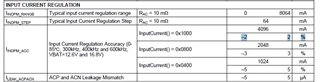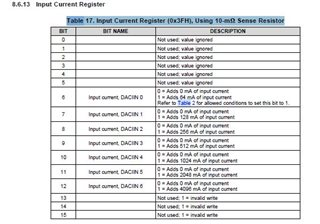If you have a related question, please click the "Ask a related question" button in the top right corner. The newly created question will be automatically linked to this question.

Part Number: BQ24800

(1).Input current limit is settted by REG 0x3FH (Condition RAC:10mohm Sense Resistor) as Chapter 8.3.5.1.
If setting the input current limit center to 4096mA(0b 0001 0000 0000 0000), it has a tolerance by +/- 2% as Electrical Characteristics as below figure.

In this case, please teach the detail of Current Regulation Accuracy +/- 2%? Is it included RAC 10mohm resistor's tolerance and Charger' s tolerance of Amplifer, etc.?

In other words, which is correct Case 1 and Case 2 of below?

Case 1. Input current limit difference is only Current Regulation Accuracy +/- 2%.

Case 2. Input current limit difference is caluculated by (Current Regulation Accuracy +/- 2%) + (RAC Sence Resistor tolerance +/- 0.5%) + (Amplifer tolerance +/- ?? %) +…

If Case 2 is corrent, we must know the above valiation items, and we must calcurate diffrenece from setting 4096mA by using valiation item's tolerance. Please teach it.(2). Max of Input current limit range is REG 0x3FH(0b 0001 1111 1100 0000)=8128mA/ RAC 10mohm Sense Resistor as Table17 as below figure.

If the input current limit:8192mA has the valiance of Current Regulation Accuracy +2% as above question (1), the actual Input current limit will be 8192mA*1.02 =8356mA?
Or, the actual current limit will be set to 8128mA by satuation even if Current Regulation Accuracy +2%?(3). Max of Input current limit range is REG 0x3FH(0b 0001 1111 1100 0000)=8128mA/ RAC 10mohm Sense Resistor as Table17.

If current comsumption is 8128mA or more at condition of RAC 10mohm, What is happened about sensing current?
For example, when current is fllowed 9000mA at RAC 10mohm, sensing value is clipped to 8128mA as max?

• Hello Norimichi,

The input current limit difference is only Current Regulation Accuracy +/-2%. This is assuming you meet all of the specifications outlined in the datasheet, if using a resistor that has a high tolerance, that tolerance will need to be added to the difference.

The maximum input current limit is 8128mA, trying to set the current higher than that will be invalid, and the value will be clipped to 8128mA as max.

Sincerely,

• Thank you for your help.

> The maximum input current limit is 8128mA, trying to set the current higher than that will be invalid, and the value will be clipped to 8128mA as max.

(4). Above crriping is by hardware?

>The input current limit difference is only Current Regulation Accuracy +/-2%.

> The maximum input current limit is 8128mA, trying to set the current higher than that will be invalid, and the value will be clipped to 8128mA as max.

(5). If set current limit to 8128mA, actual current limit is 8128mA or less even if condition is current regulation accuracy + 2%, is it correct? Or even if  set current limit to 8128mA, actual current limit might be 8128mA or more by tolerance of current regulation accuracy + 2%? (e.g. 8290mA)

(6). When condition of RAC 5mohm, maximum measurement input current is 16256mA. If actual input current consumption is higher than 16256mA by peak    current , for example 23000mA, etc,
is input current limit behavior no problem?
We affraid that abnormal result will been occurred by reaching overflow from 16256mA , etc.

Best regards,

• Today is a US holiday, please check it back after Tuesday.

• Hello Norimichi,

The limit is set by programming the input current limit register to the desired value. The device will not reach an input current measurement higher than what the limit has been set to. You cannot set current limit to be higher than 8128mA, and the actual current limit will not exceed 8128mA, it could be less based n the current regulation accuracy. For RAC 5mOhm, the maximum input current is 16256mA, actual input current will not exceed 16256mA, it will be limited to that value and the device will not exceed 16256mA.

Sincerely,

• Thank you for your help.

We would like to confrm just to make sure.

When Input Current limit REG 0x3Fh is set to 8128mA max, and input current sensing resistor RAC is set to 5m ohm,

Input Current limit actual operating points is 16256mA +/- 2%?

Is it correct? (+/- 2% is accuracy)

Best regards,

• Hello Norimichi,

This is correct.

Sincerely,